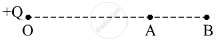# A Point Charge +Q is Placed at Point O, as Shown in the Figure. is the Potential Difference Va – Vb Positive, Negative Or Zero? - Physics

A point charge +Q is placed at point O, as shown in the figure. Is the potential difference VAVB positive, negative or zero?#### Solution

Electric potential at a distance r from the point charge +Q is given by

V(r) = 1/(4πε_0) Q/R

Potential at point A will be

V(r_A) = 1/(4πε_0) Q/r_A

Similarly, potential at point B will be

V(r_B) = 1/(4πε_0) Q/r_A

because r_A < r_B

therefore V_A > V_B

therefore V_A - V_B > 0

Concept: Potential Due to a Point Charge
Is there an error in this question or solution?
2015-2016 (March) Delhi Set 1

Share